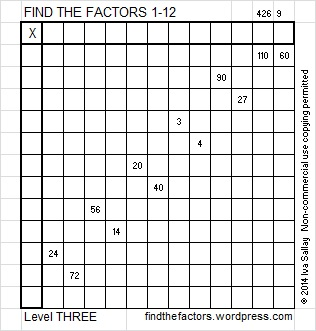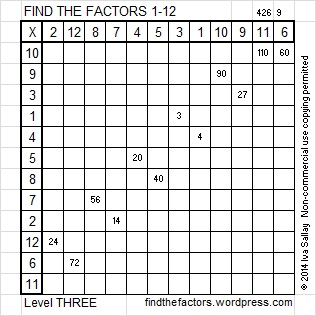# 163 and Level 3

• 163 is a prime number.
• Prime factorization: 163 is prime.
• The exponent of prime number 163 is 1. Adding 1 to that exponent we get (1 + 1) = 2. Therefore 163 has exactly 2 factors.
• Factors of 163: 1, 163
• Factor pairs: 163 = 1 x 163
• 163 has no square factors that allow its square root to be simplified. √163 ≈ 12.7671How do we know that 163 is a prime number? If 163 were not a prime number, then it would be divisible by at least one prime number less than or equal to √163 ≈ 12.8. Since 163 cannot be divided evenly by 2, 3, 5, 7, or 11, we know that 163 is a prime number.Excel file of puzzles and previous week’s factor solutions: 12 Factors 2014-06-30

A Logical Approach to FIND THE FACTORS: Find the column or row with two clues and find their common factor. Write the corresponding factors in the factor column (1st column) and factor row (top row).  Because this is a level three puzzle, you have now written a factor at the top of the factor column. Continue to work from the top of the factor column to the bottom, finding factors and filling in the factor column and the factor row one cell at a time as you go.## 2 thoughts on “163 and Level 3”

1.Paula Beardell Krieg

Ah! I love that you wrote what you did about how to know that 163 is prime! I’ve wondered a bit about this sort of thing, but assumed that it would be way to complex to absorb. I am delighted to have been wrong. thank you.

•ivasallay

You’re quite welcome!

This site uses Akismet to reduce spam. Learn how your comment data is processed.